# Selina Solutions Concise Maths Class 10 Chapter 20 Cylinder, Cone and Sphere (Surface Area and Volume) Exercise 20(A)

A solid which has a uniform circular cross-section, is called a cylinder. In this exercise, students will be tested to find the surface area and volume of cylinders and hollow cylinders. For students wishing to learn the right steps of solving such problems, Selina Solutions for Class 10 Maths is the right resource for you. The solutions to the Concise Selina Solutions for Class 10 Maths Chapter 20 Cylinder, Cone and Sphere (Surface Area and Volume) Exercise 20(A) are accessible in PDF format, provided in the link below.

## Selina Solutions Concise Maths Class 10 Chapter 20 Cylinder, Cone and Sphere (Surface Area and Volume) Exercise 20(A) Download PDF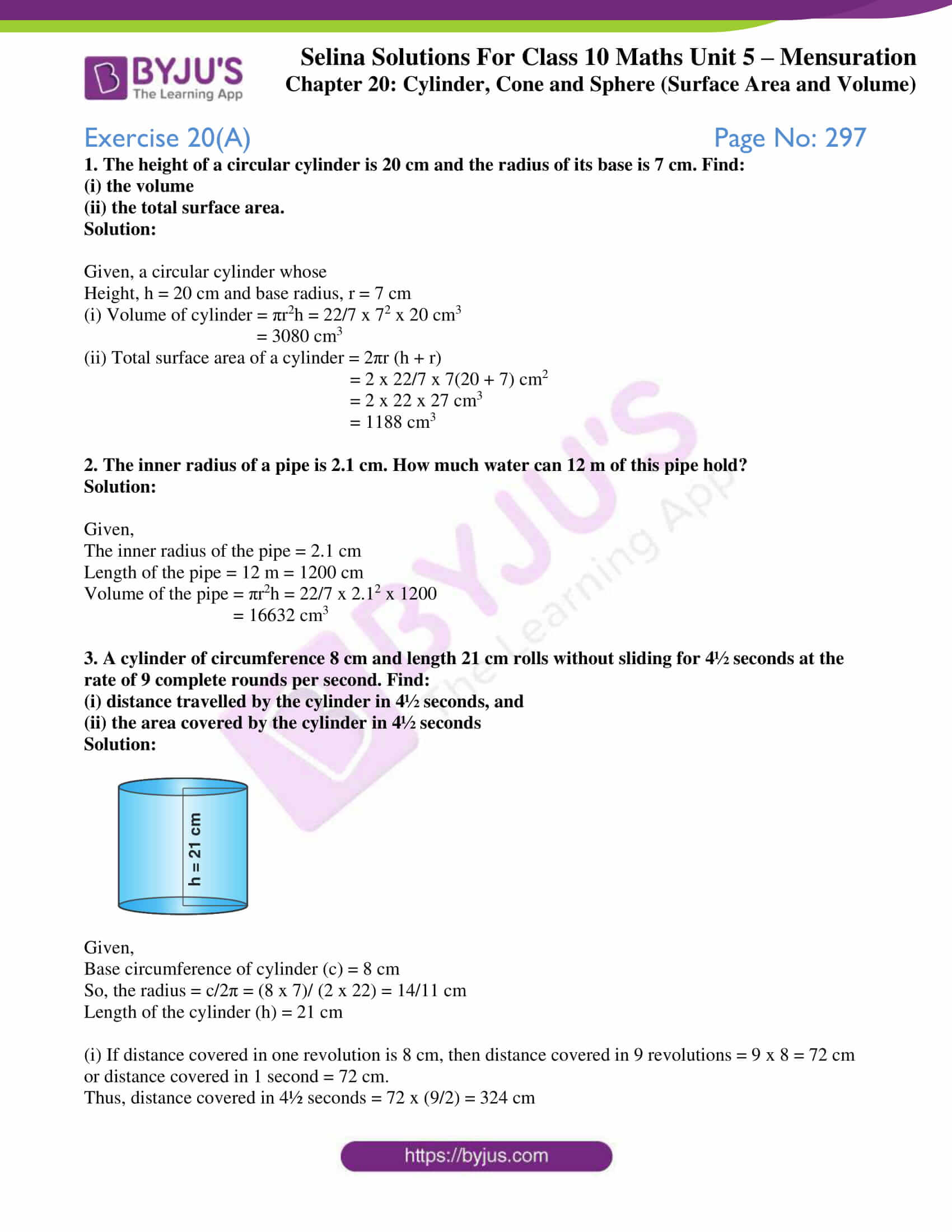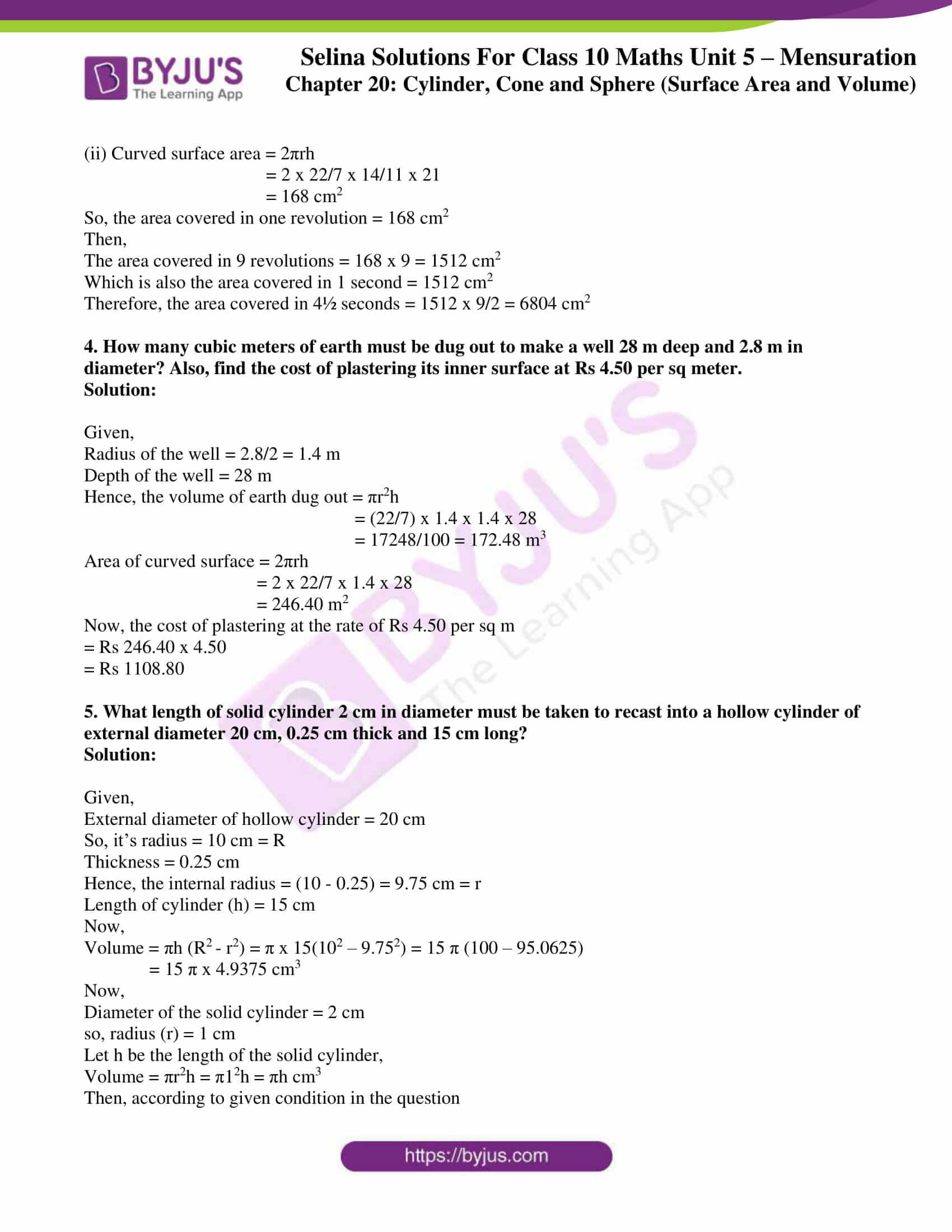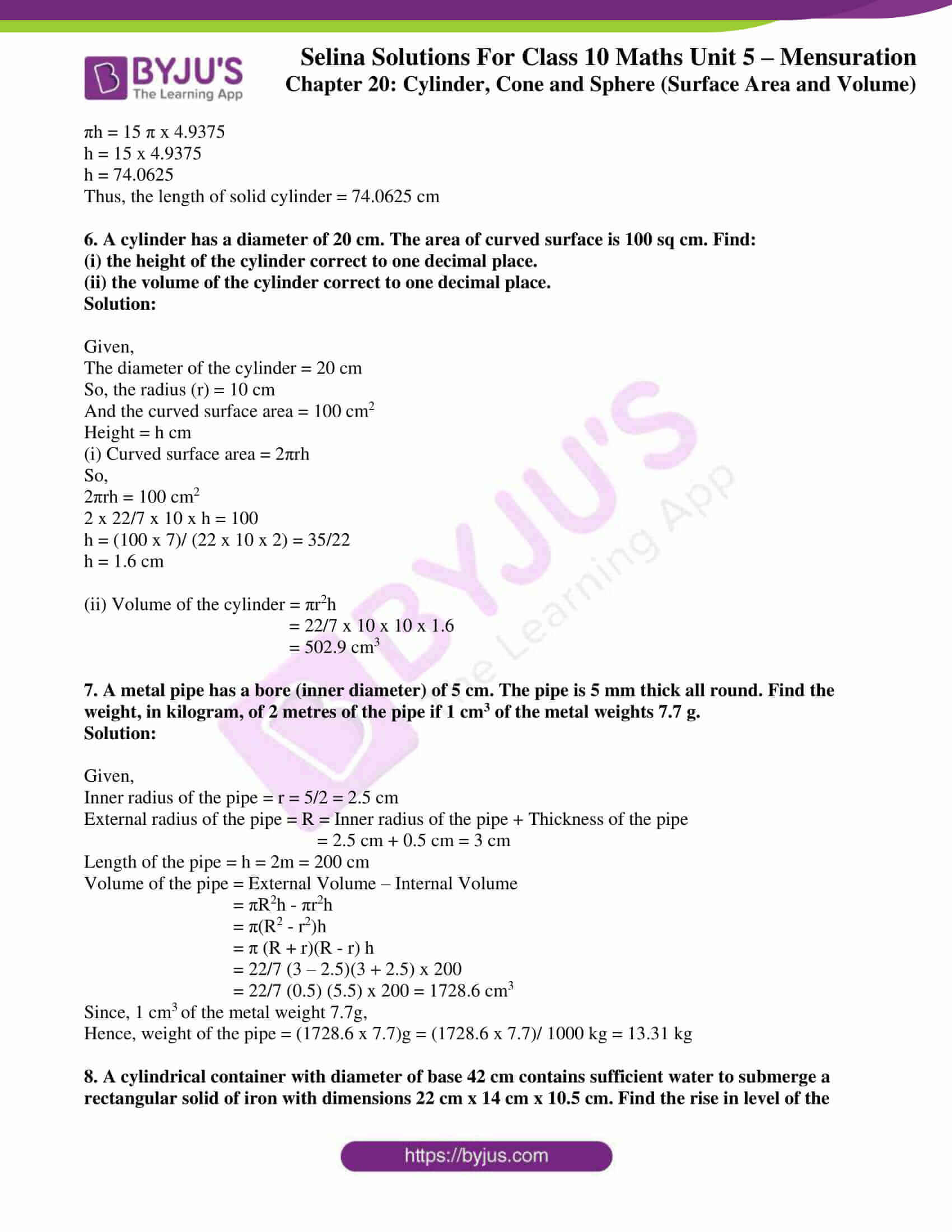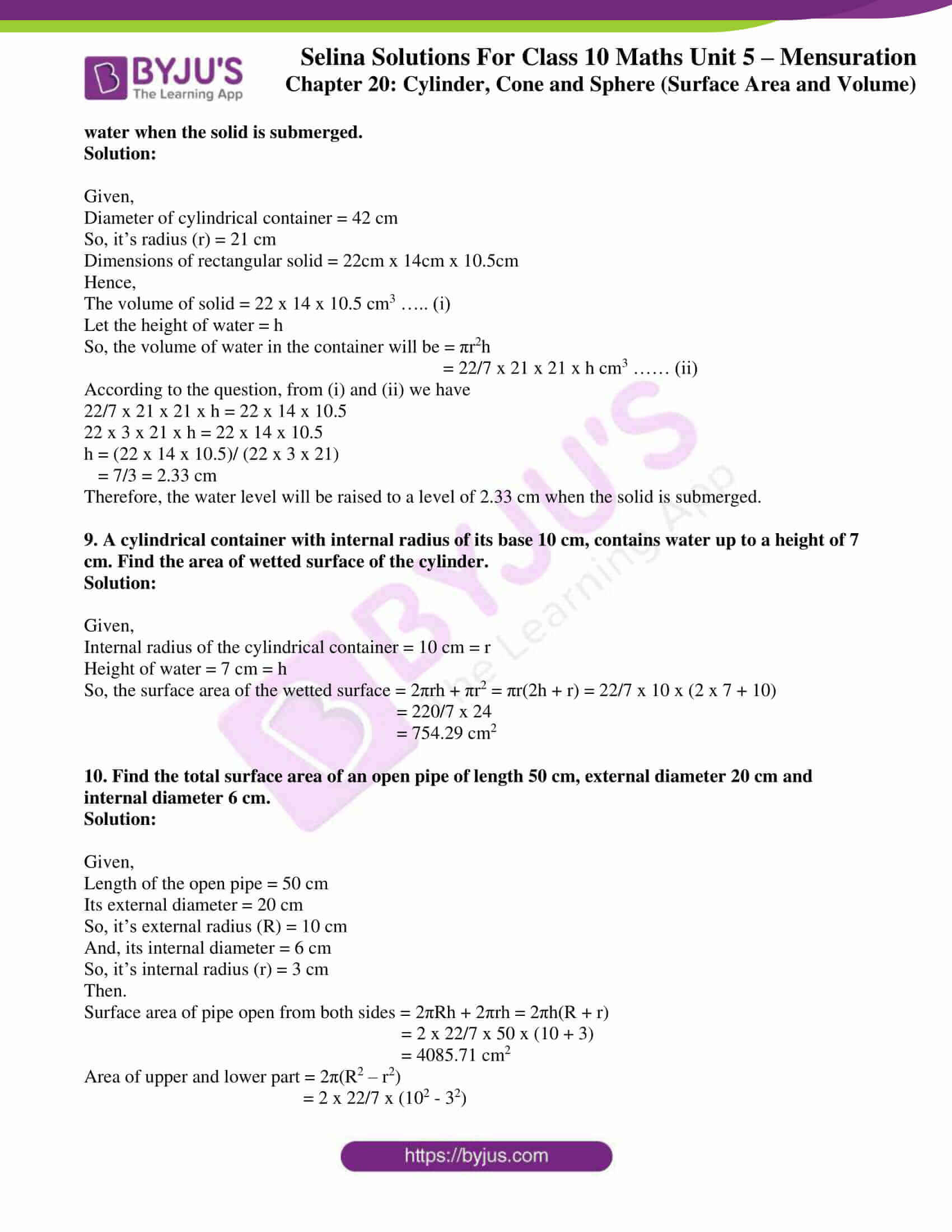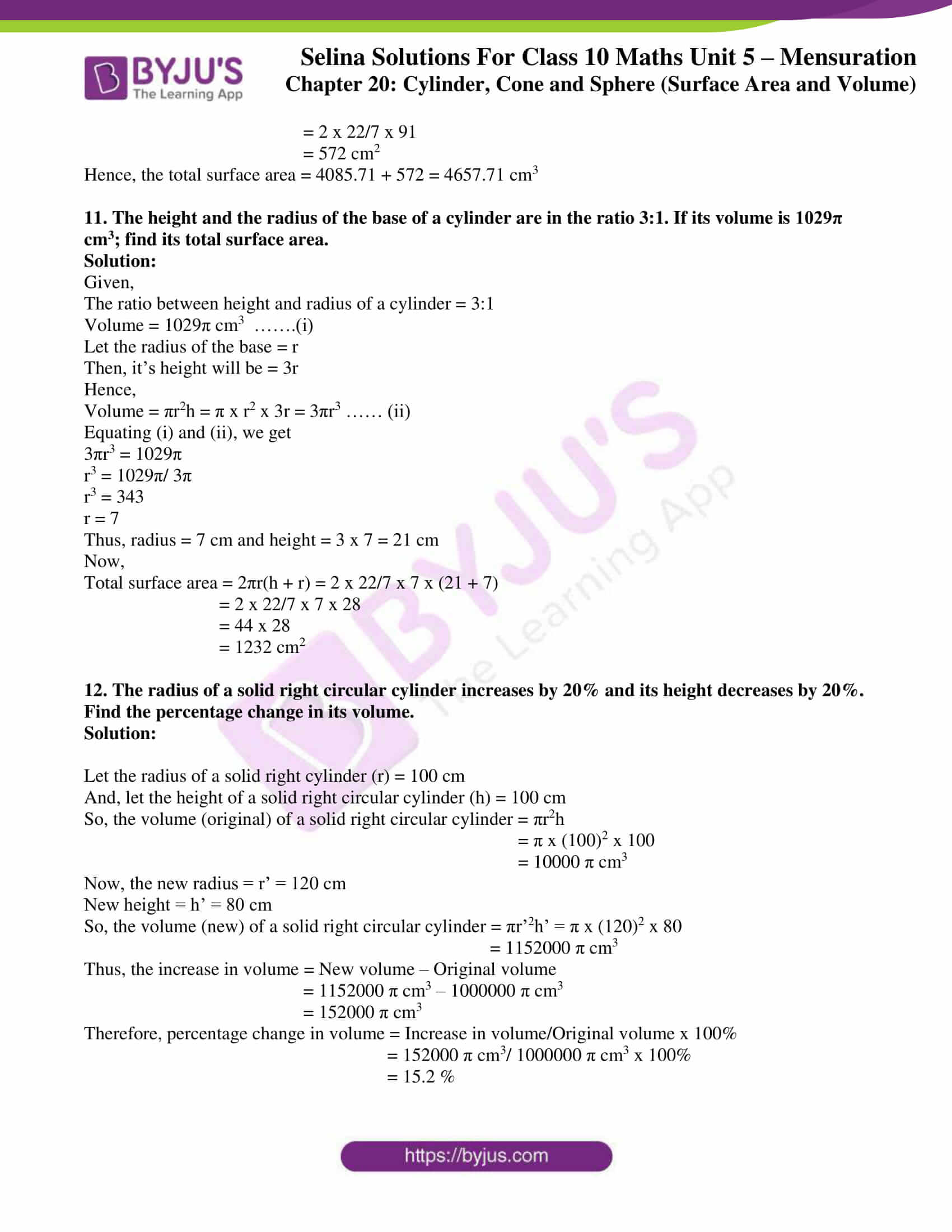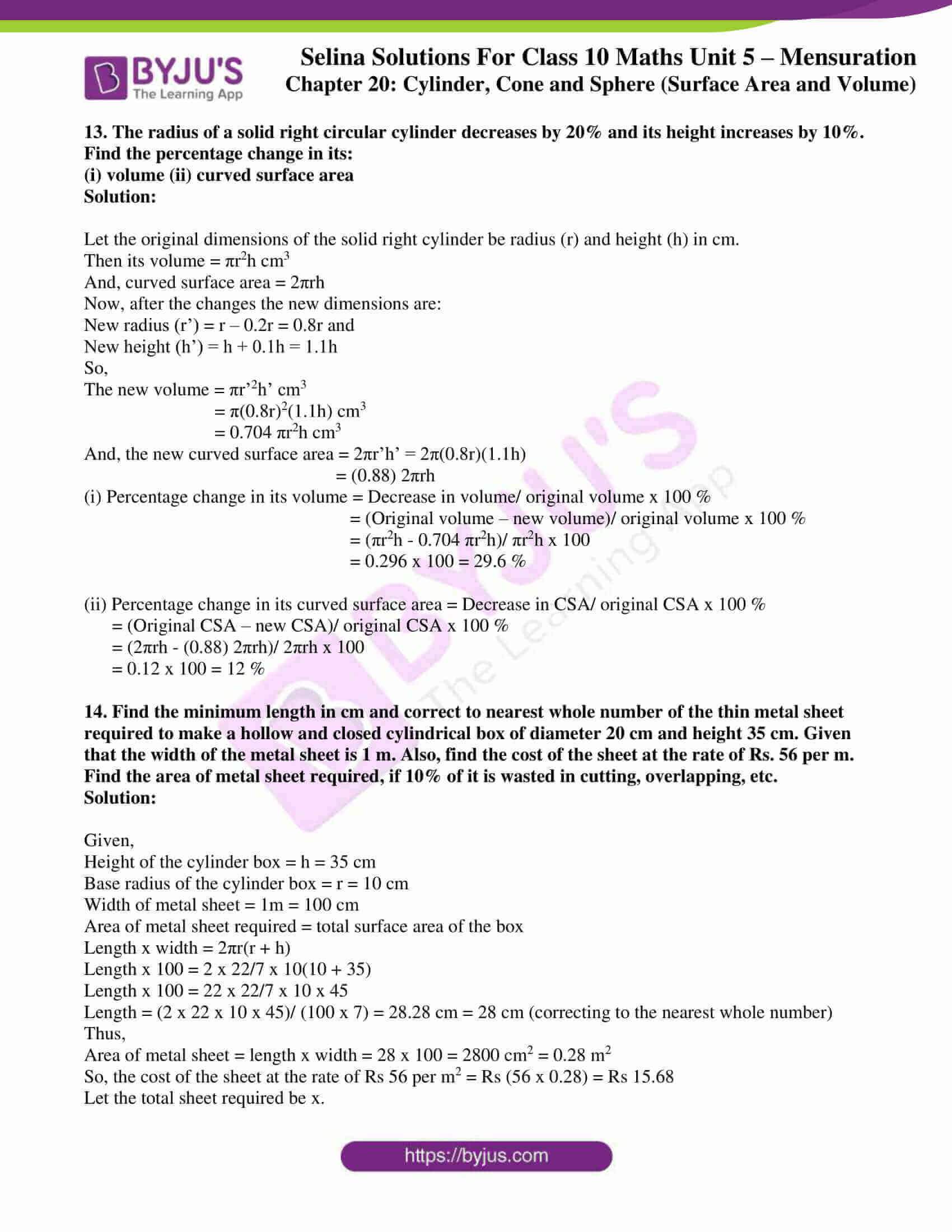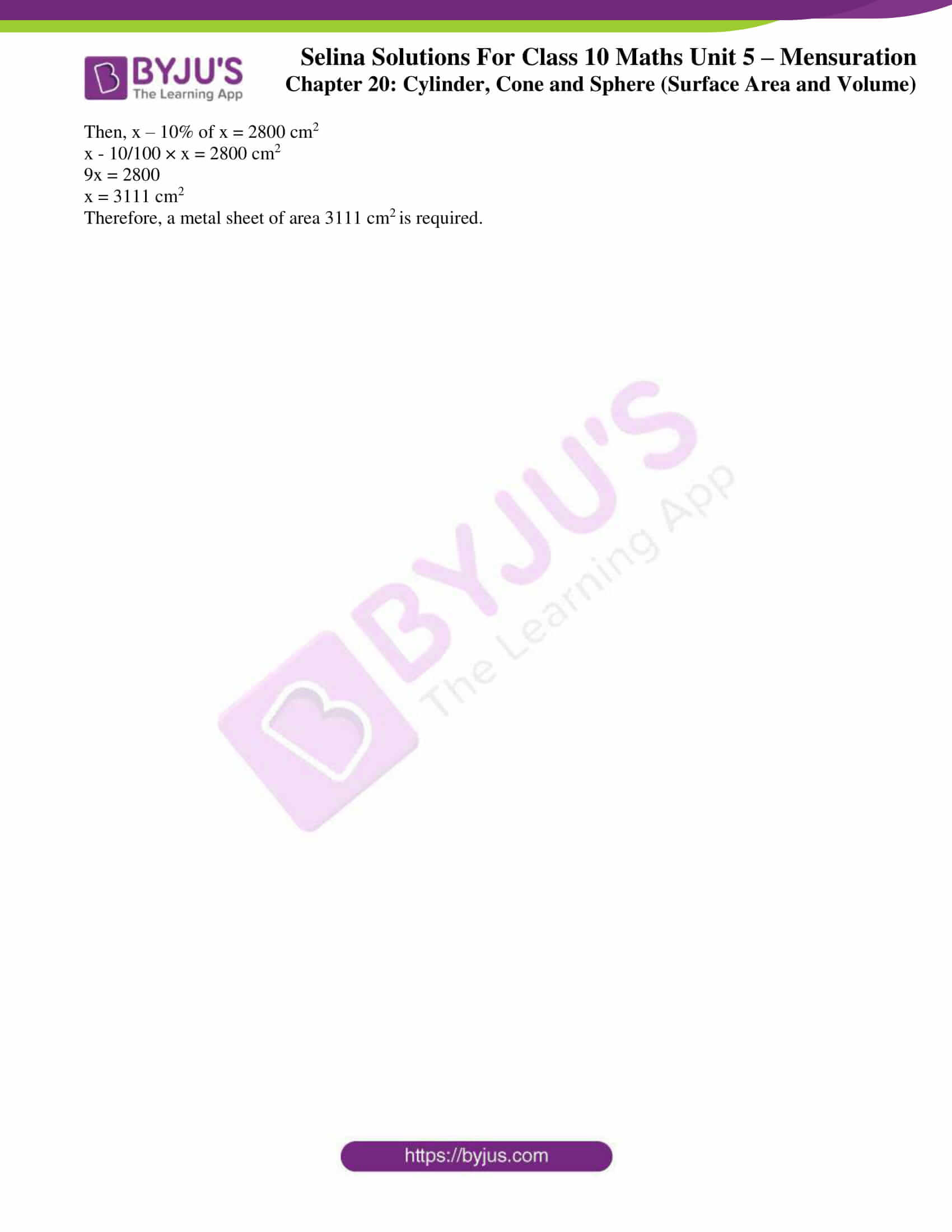### Access Selina Solutions Concise Maths Class 10 Chapter 20 Cylinder, Cone and Sphere (Surface Area and Volume) Exercise 20(A)

1. The height of a circular cylinder is 20 cm and the radius of its base is 7 cm. Find:

(i) the volume

(ii) the total surface area.

Solution:

Given, a circular cylinder whose

Height, h = 20 cm and base radius, r = 7 cm

(i) Volume of cylinder = πr2h = 22/7 x 72 x 20 cm3

= 3080 cm3

(ii) Total surface area of a cylinder = 2πr (h + r)

= 2 x 22/7 x 7(20 + 7) cm2

= 2 x 22 x 27 cm3

= 1188 cm3

2. The inner radius of a pipe is 2.1 cm. How much water can 12 m of this pipe hold?

Solution:

Given,

The inner radius of the pipe = 2.1 cm

Length of the pipe = 12 m = 1200 cm

Volume of the pipe = πr2h = 22/7 x 2.12 x 1200

= 16632 cm3

3. A cylinder of circumference 8 cm and length 21 cm rolls without sliding for 4½ seconds at the rate of 9 complete rounds per second. Find:

(i) distance travelled by the cylinder in 4½ seconds, and

(ii) the area covered by the cylinder in 4½ seconds

Solution: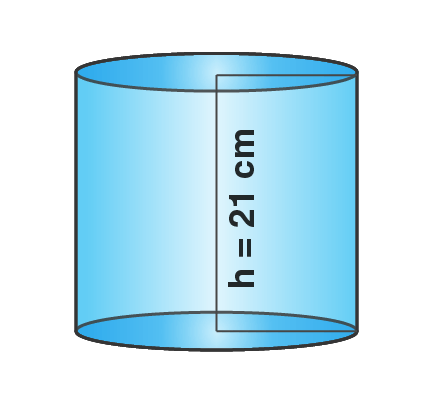Given,

Base circumference of cylinder (c) = 8 cm

So, the radius = c/2π = (8 x 7)/ (2 x 22) = 14/11 cm

Length of the cylinder (h) = 21 cm

(i) If distance covered in one revolution is 8 cm, then distance covered in 9 revolutions = 9 x 8 = 72 cm or distance covered in 1 second = 72 cm.

Thus, distance covered in 4½ seconds = 72 x (9/2) = 324 cm

(ii) Curved surface area = 2πrh

= 2 x 22/7 x 14/11 x 21

= 168 cm2

So, the area covered in one revolution = 168 cm2

Then,

The area covered in 9 revolutions = 168 x 9 = 1512 cm2

Which is also the area covered in 1 second = 1512 cm2

Therefore, the area covered in 4½ seconds = 1512 x 9/2 = 6804 cm2

4. How many cubic meters of earth must be dug out to make a well 28 m deep and 2.8 m in diameter? Also, find the cost of plastering its inner surface at Rs 4.50 per sq meter.

Solution:

Given,

Radius of the well = 2.8/2 = 1.4 m

Depth of the well = 28 m

Hence, the volume of earth dug out = πr2h

= (22/7) x 1.4 x 1.4 x 28

= 17248/100 = 172.48 m3

Area of curved surface = 2πrh

= 2 x 22/7 x 1.4 x 28

= 246.40 m2

Now, the cost of plastering at the rate of Rs 4.50 per sq m

= Rs 246.40 x 4.50

= Rs 1108.80

5. What length of solid cylinder 2 cm in diameter must be taken to recast into a hollow cylinder of external diameter 20 cm, 0.25 cm thick and 15 cm long?

Solution:

Given,

External diameter of hollow cylinder = 20 cm

So, it’s radius = 10 cm = R

Thickness = 0.25 cm

Hence, the internal radius = (10 – 0.25) = 9.75 cm = r

Length of cylinder (h) = 15 cm

Now,

Volume = πh (R2 – r2) = π x 15(102 – 9.752) = 15 π (100 – 95.0625)

= 15 π x 4.9375 cm3

Now,

Diameter of the solid cylinder = 2 cm

so, radius (r) = 1 cm

Let h be the length of the solid cylinder,

Volume = πr2h = π12h = πh cm3

Then, according to given condition in the question

πh = 15 π x 4.9375

h = 15 x 4.9375

h = 74.0625

Thus, the length of solid cylinder = 74.0625 cm

6. A cylinder has a diameter of 20 cm. The area of curved surface is 100 sq cm. Find:

(i) the height of the cylinder correct to one decimal place.

(ii) the volume of the cylinder correct to one decimal place.

Solution:

Given,

The diameter of the cylinder = 20 cm

So, the radius (r) = 10 cm

And the curved surface area = 100 cm2

Height = h cm

(i) Curved surface area = 2πrh

So,

2πrh = 100 cm2

2 x 22/7 x 10 x h = 100

h = (100 x 7)/ (22 x 10 x 2) = 35/22

h = 1.6 cm

(ii) Volume of the cylinder = πr2h

= 22/7 x 10 x 10 x 1.6

= 502.9 cm3

7. A metal pipe has a bore (inner diameter) of 5 cm. The pipe is 5 mm thick all round. Find the weight, in kilogram, of 2 metres of the pipe if 1 cm3 of the metal weights 7.7 g.

Solution:

Given,

Inner radius of the pipe = r = 5/2 = 2.5 cm

External radius of the pipe = R = Inner radius of the pipe + Thickness of the pipe

= 2.5 cm + 0.5 cm = 3 cm

Length of the pipe = h = 2m = 200 cm

Volume of the pipe = External Volume – Internal Volume

= πR2h – πr2h

= π(R2 – r2)h

= π (R + r)(R – r) h

= 22/7 (3 – 2.5)(3 + 2.5) x 200

= 22/7 (0.5) (5.5) x 200 = 1728.6 cm3

Since, 1 cm3 of the metal weight 7.7g,

Hence, weight of the pipe = (1728.6 x 7.7)g = (1728.6 x 7.7)/ 1000 kg = 13.31 kg

8. A cylindrical container with diameter of base 42 cm contains sufficient water to submerge a rectangular solid of iron with dimensions 22 cm x 14 cm x 10.5 cm. Find the rise in level of the water when the solid is submerged.

Solution:

Given,

Diameter of cylindrical container = 42 cm

So, it’s radius (r) = 21 cm

Dimensions of rectangular solid = 22cm x 14cm x 10.5cm

Hence,

The volume of solid = 22 x 14 x 10.5 cm3 ….. (i)

Let the height of water = h

So, the volume of water in the container will be = πr2h

= 22/7 x 21 x 21 x h cm3 …… (ii)

According to the question, from (i) and (ii) we have

22/7 x 21 x 21 x h = 22 x 14 x 10.5

22 x 3 x 21 x h = 22 x 14 x 10.5

h = (22 x 14 x 10.5)/ (22 x 3 x 21)

= 7/3 = 2.33 cm

Therefore, the water level will be raised to a level of 2.33 cm when the solid is submerged.

9. A cylindrical container with internal radius of its base 10 cm, contains water up to a height of 7 cm. Find the area of wetted surface of the cylinder.

Solution:

Given,

Internal radius of the cylindrical container = 10 cm = r

Height of water = 7 cm = h

So, the surface area of the wetted surface = 2πrh + πr2 = πr(2h + r) = 22/7 x 10 x (2 x 7 + 10)

= 220/7 x 24

= 754.29 cm2

10. Find the total surface area of an open pipe of length 50 cm, external diameter 20 cm and internal diameter 6 cm.

Solution:

Given,

Length of the open pipe = 50 cm

Its external diameter = 20 cm

So, it’s external radius (R) = 10 cm

And, its internal diameter = 6 cm

So, it’s internal radius (r) = 3 cm

Then.

Surface area of pipe open from both sides = 2πRh + 2πrh = 2πh(R + r)

= 2 x 22/7 x 50 x (10 + 3)

= 4085.71 cm2

Area of upper and lower part = 2π(R2 – r2)

= 2 x 22/7 x (102 – 32)

= 2 x 22/7 x 91

= 572 cm2

Hence, the total surface area = 4085.71 + 572 = 4657.71 cm3

11. The height and the radius of the base of a cylinder are in the ratio 3:1. If its volume is 1029π cm3; find its total surface area.

Solution:

Given,

The ratio between height and radius of a cylinder = 3:1

Volume = 1029π cm3  …….(i)

Let the radius of the base = r

Then, it’s height will be = 3r

Hence,

Volume = πr2h = π x r2 x 3r = 3πr3 …… (ii)

Equating (i) and (ii), we get

3πr3 = 1029π

r3 = 1029π/ 3π

r3 = 343

r = 7

Thus, radius = 7 cm and height = 3 x 7 = 21 cm

Now,

Total surface area = 2πr(h + r) = 2 x 22/7 x 7 x (21 + 7)

= 2 x 22/7 x 7 x 28

= 44 x 28

= 1232 cm2

12. The radius of a solid right circular cylinder increases by 20% and its height decreases by 20%. Find the percentage change in its volume.

Solution:

Let the radius of a solid right cylinder (r) = 100 cm

And, let the height of a solid right circular cylinder (h) = 100 cm

So, the volume (original) of a solid right circular cylinder = πr2h

= π x (100)2 x 100

= 10000 π cm3

Now, the new radius = r’ = 120 cm

New height = h’ = 80 cm

So, the volume (new) of a solid right circular cylinder = πr’2h’ = π x (120)2 x 80

= 1152000 π cm3

Thus, the increase in volume = New volume – Original volume

= 1152000 π cm3 – 1000000 π cm

= 152000 π cm3

Therefore, percentage change in volume = Increase in volume/Original volume x 100%

= 152000 π cm3/ 1000000 π cm3 x 100%

= 15.2 %

13. The radius of a solid right circular cylinder decreases by 20% and its height increases by 10%. Find the percentage change in its:

(i) volume (ii) curved surface area

Solution:

Let the original dimensions of the solid right cylinder be radius (r) and height (h) in cm.

Then its volume = πr2h cm3

And, curved surface area = 2πrh

Now, after the changes the new dimensions are:

New radius (r’) = r – 0.2r = 0.8r and

New height (h’) = h + 0.1h = 1.1h

So,

The new volume = πr’2h’ cm3

= π(0.8r)2(1.1h) cm3

= 0.704 πr2h cm3

And, the new curved surface area = 2πr’h’ = 2π(0.8r)(1.1h)

= (0.88) 2πrh

(i) Percentage change in its volume = Decrease in volume/ original volume x 100 %

= (Original volume – new volume)/ original volume x 100 %

= (πr2h – 0.704 πr2h)/ πr2h x 100

= 0.296 x 100 = 29.6 %

(ii) Percentage change in its curved surface area = Decrease in CSA/ original CSA x 100 %

= (Original CSA – new CSA)/ original CSA x 100 %

= (2πrh – (0.88) 2πrh)/ 2πrh x 100

= 0.12 x 100 = 12 %

14. Find the minimum length in cm and correct to nearest whole number of the thin metal sheet required to make a hollow and closed cylindrical box of diameter 20 cm and height 35 cm. Given that the width of the metal sheet is 1 m. Also, find the cost of the sheet at the rate of Rs. 56 per m.

Find the area of metal sheet required, if 10% of it is wasted in cutting, overlapping, etc.

Solution:

Given,

Height of the cylinder box = h = 35 cm

Base radius of the cylinder box = r = 10 cm

Width of metal sheet = 1m = 100 cm

Area of metal sheet required = total surface area of the box

Length x width = 2πr(r + h)

Length x 100 = 2 x 22/7 x 10(10 + 35)

Length x 100 = 22 x 22/7 x 10 x 45

Length = (2 x 22 x 10 x 45)/ (100 x 7) = 28.28 cm = 28 cm (correcting to the nearest whole number)

Thus,

Area of metal sheet = length x width = 28 x 100 = 2800 cm2 = 0.28 m2

So, the cost of the sheet at the rate of Rs 56 per m2 = Rs (56 x 0.28) = Rs 15.68

Let the total sheet required be x.

Then, x – 10% of x = 2800 cm2

x – 10/100 × x = 2800 cm2

9x = 2800

x = 3111 cm2

Therefore, a metal sheet of area 3111 cm2 is required.